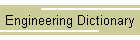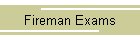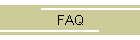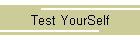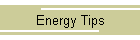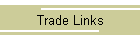## Article-The Rankine Cycle

Energy Conservation in Industry, Combustion, Heat Recovery and Rankine Cycle MachinesRankine cycle is a heat engine with vapor power cycle. The common working fluid is water. The cycle consists of four processes:Work output of the cycle (Steam turbine), W1 and work input to the cycle (Pump), W2 are:

```	W1 = m (h1-h2)
W2 = m (h4-h3)
```

where m is the mass flow of the cycle. Heat supplied to the cycle (boiler), Q1 and heat rejected from the cycle (condenser), Q2 are:

```	Q1 = m (h1-h4)
Q2 = m (h2-h3)
```

The net work output of the cycle is:

```	W = W1 - W2
```

The thermal efficiency of a Rankine cycle is: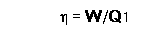The efficiency of the Rankine cycle is not as high as Carnot cycle but the cycle has less practical difficulties and more economic.